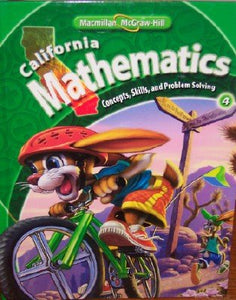California Mathematics Grade 4 (Student Edition: Concepts, Skills, And Problem Solving)

• Publish Date: 2009-12-21
• Binding: Hardcover
• Author: Mary Behr Altieri
Regular price \$44.26 Sale price \$15.43

Attention: For textbook, access codes and supplements are not guaranteed with used items.

ch 1: Place Value and Number Sense. ch 2: Addition and Subtraction. ch 3: Algebra: Use Addition and Subtraction. ch 4: Statistics: Data and Graphs. ch 5: Multiplication and Division Facts. ch 6: Algebra: Use Multiplication and Division. ch 7: Multiply by one-digit Numbers. ch 8: Multiply by two-digit Numbers. ch 9: Divide by one-digit Numbers. ch 10: Geometry. ch 11: Geometry and Measurement. ch 12: Algebra and Graphing. ch 13: Fractions. ch 14: Decimals. ch 15: Decimals: Additon and Subtraction. 16: probability. Standards Review. 681 pages.